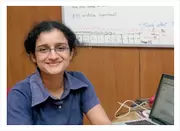X
Low dimensional modeling of combustion in spark ignition engines
Published in SAE International
2013
Volume: 5

Abstract
Engine modelling aims at studying the combustion related phenomenon occurring in Internal Combustion (IC) engines. In this regard, a low dimensional mathematical model using first principles has been developed to study Spark Ignited (SI) engines. The resulting equations are Ordinary Differential Equations (ODE) (for volume, pressure, torque, speed and work done) and Partial Differential Equations (PDEs) for temperature and species conservation equations (fuel, CO, CO2, NO). This model utilizes simplified reaction kinetics for the oxidation of fuel in the combustion chamber. A two-step mechanism for the combustion of fuel and the classical Zeldovich Mechanism are used to predict the amount of NO formed during combustion. The model is solved in FORTRAN using LSODE subroutine (for stiff equations) with lumped parameters for thermal properties and diffusion, and invoking the ideal gas assumption. Method of Lines (MOL) has been used to study the axial and transient variation of parameters such as temperature and composition of the exhaust. With fuel composition, RPM, physical engine parameters and amount of fuel as inputs, the model captures the in-cylinder variation of pressure, temperature, exit gas composition (CO, CO2, octane, NO, O2) and work done by the piston with respect to crank angle. The results are comparable qualitatively with experimental data. The simulated data is validated against experimental data of pressure variation versus crank angle and mass fraction of the burned species. Copyright © 2013 SAE International and Copyright © 2013 SIAT, India.
Journal SAE Technical Papers SAE International 01487191 No
Authors (1)
•Concepts (19)
•ANALOG DIFFERENTIAL ANALYZERS
•Association reactions
•Carbon dioxide
•Combustion chambers
•Compression ratio (machinery)
•Conservation
•Engine cylinders
•Fuels
•Ordinary differential equations
•Reaction kinetics
•Crank angle
•Engine modelling
•LOW-DIMENSIONAL MODELING
•Ordinary differential equation (ode)
•Partial differential equations (pdes)
•SPARK-IGNITED ENGINES
•SPECIES CONSERVATIONS
•VARIATION OF PARAMETERS
•Ignition